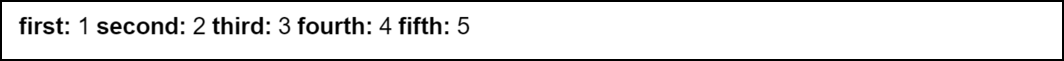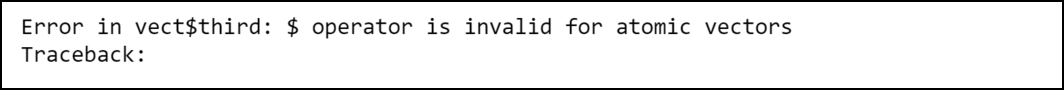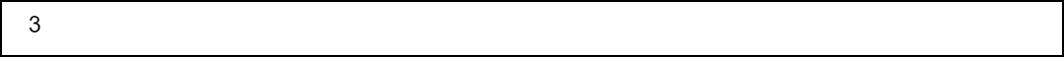GFG App
Open AppBrowser
Continue

# How to Fix in R: \$ operator is invalid for atomic vectors

In this article, we are going to see how to fix \$ operator is invalid for atomic vectors in the R programming language.

The error that one may face in R is:

`\$ operator is invalid for atomic vectors`

Such an error is produced by the R compiler when we try to get an element of an atomic vector by using the \$ operator. An atomic vector is simply a 1-dimensional object containing data created with the help of c() and vector() functions. R doesn’t allow us to access elements of an atomic vector using the \$ symbol. But we can use double brackets i.e, [[]] or the getElement() function to access them.

## When this error might occur

Let’s consider an example in which we have a numeric vector vect, initialized with the first five natural numbers. Each of the numbers is assigned with a name using the names() function in R. The syntax of the names() function is given below:

Syntax: names(vect) <- value

Parameters:

• vect: an Object i.e. vector, matrix, data frame, etc.
• value: The corresponding names to be assigned

## R

 `# Define a vector ` `vect <- ``c``(1, 2, 3, 4, 5) ` ` `  `# Set integers names ` `names``(vect) <- ``c``(``'first'``, ``'second'``, ``'third'``, ` `                 ``'fourth'``, ``'fifth'``) ` ` `  `# Display the vector ` `vect`

Output:Now let’s try to access the element third element using the statement, vect\$third:

## R

 `# Define a vector ` `vect <- ``c``(1, 2, 3, 4, 5) ` ` `  `# Set integers names ` `names``(vect) <- ``c``(``'first'``, ``'second'``, ``'third'``, ` `                 ``'fourth'``, ``'fifth'``) ` ` `  `# Display the vector ` `vect\$third`

Output:The R compiler produces an error because we are not allowed to access elements in an atomic vector this way. To check whether the vector is really an atomic vector, we can use is.atomic() function in R. The syntax of this function is given below:

Syntax: is.atomic(vect)

Parameters:

vect: Here, vect represents a vector

Return Type:

• TRUE: If vect is an atomic vector
• FALSE: If vect is not an atomic vector

## How to fix the error

There are three ways using which we can fix this error:

### Method 1: Accessing elements using double brackets

We can access elements of an atomic vector easily with the help of double brackets that it, [[]]:

## R

 `# Define a vector ` `vect <- ``c``(1, 2, 3, 4, 5) ` ` `  `# Set integers names ` `names``(vect) <- ``c``(``'first'``, ``'second'``, ``'third'``,  ` `                 ``'fourth'``, ``'fifth'``) ` ` `  `# Display the third element of the  ` `# vector ` `vect[[``'third'``]]`

Output:### Method 2: Accessing elements using getElement() function

Another way is to use the getElement() function to access elements of the atomic vector. The function has the following syntax:

Syntax: getElement(vect, specifier)

Parameters:

• vect: It represents a vector
• specifier: It represent the name of the element that we want to access

Return Type:

• Returns an element marked with specifier

## R

 `# Define a vector ` `vect <- ``c``(1, 2, 3, 4, 5) ` ` `  `# Set integers names ` `names``(vect) <- ``c``(``'first'``, ``'second'``, ``'third'``,  ` `                 ``'fourth'``, ``'fifth'``) ` ` `  `# Display the third element of  ` `# the vector ` `getElement``(vect, ``'third'``)`

Output:### Method 3: Accessing elements by converting the vector to data frame & then using \$ operator

The other way is to convert the vector to a data frame firstly and then apply the \$ operator. We can convert a vector into a data frame using as.data.frame() function. The syntax of this function is given below:

Syntax: as.data.frame(object)

Parameter:

• object represents a vector or matrix

Return Type:

• Returns a vector

## R

 `# Define a vector ` `vect <- ``c``(1, 2, 3, 4, 5) ` ` `  `# Set integers names ` `names``(vect) <- ``c``(``'first'``, ``'second'``, ``'third'``,  ` `                 ``'fourth'``, ``'fifth'``) ` ` `  `# Transform the vector to data frame ` `dataframe <- ``as.data.frame``(``t``(vect)) ` ` `  `# Access the third element ` `dataframe\$third`

Output:My Personal Notes arrow_drop_up